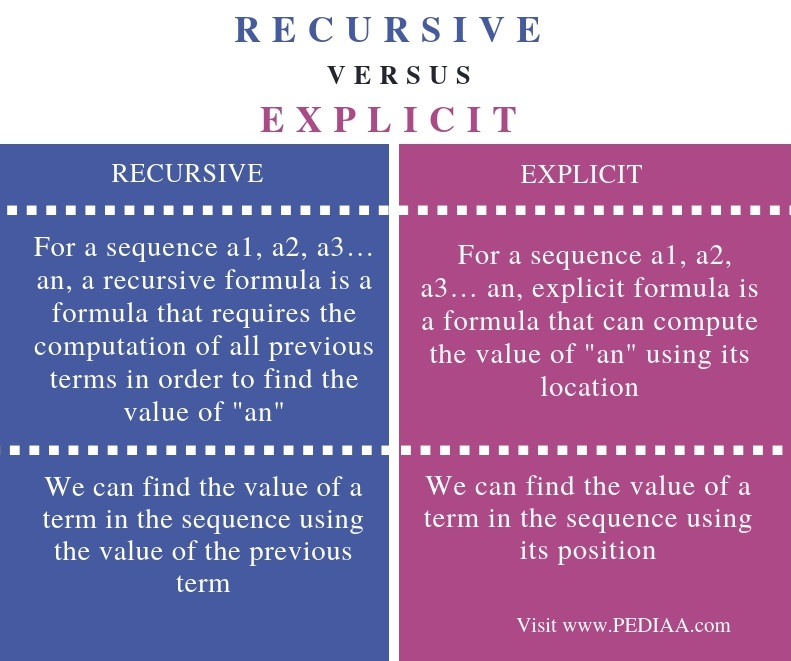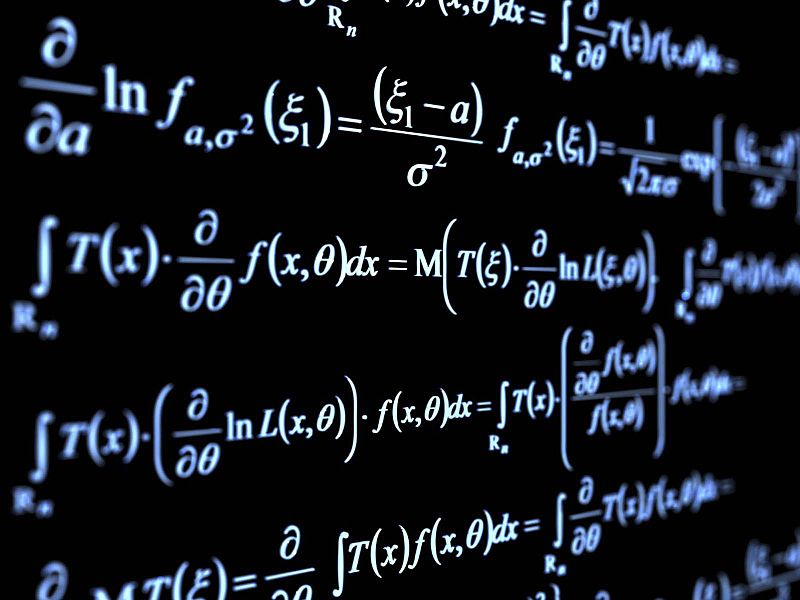# What is the Difference Between Recursive and Explicit

The main difference between recursive and explicit is that a recursive formula gives the value of a specific term based on the previous term while an explicit formula gives the value of a specific term based on the position.

A sequence is an important concept in mathematics. It refers to a set of numbers placed in order. We can represent an arithmetic sequence using a formula. In other words, we can directly compute any term of the sequence using a formula. There are two types of formulas as recursive and explicit formulas. A formula describes a way of finding any term in the sequence.

### Key Areas Covered

1. What is Recursive
– Definition, Functionality
2. What is Explicit
– Definition, Functionality
3. Difference Between Recursive and Explicit
– Comparison of Key Differences

### Key Terms

Explicit Formula, Recursive Formula## What is Recursive

In a recursive formula, we can find the value of a specific term based on the previous term.

For example, assume a formula as follows.

a(n) = a(n-1) +5

The first term of the sequence is a(1)=3

The second term is as follows.

a(2) = a(2-1) + 5

a(2) = a(1) + 5

We can substitute value to the above formula.  Then it will give the result for a(2).

a(2) = 3 + 5

a(2) = 8

Similarly, we can find the third term as follows.

a(3) = a(2) + 5

a(3) = 8+5 = 13

Calculating fourth term is as follows.

a(4) = a(3) + 5

a(4) = 13 + 5 = 18Likewise, we can calculate the values of the terms in the sequence. To find a (4), we need the value of a(3). To find a (3), we need the value of a (2) and to find the value a (2), we need the value of a(1). Therefore, it requires the previous term or terms to find the value of a specific term. That is the functionality of recursive formulas.

## What is Explicit

In explicit formulas, we can find the value of a specific term based on its position.

Assume a formula as follows.

a(n) = 2(n-1) + 4

First term is as follows.

a(1) = 2 (1-1) + 4 = 0 + 4 = 4

Second term is as follows.

a(2) = 2(2-1) + 4 = 2+4 = 6

Third term is as follows.

a(3) = 2(3-1) + 4 = 4 +4 = 8

Fourth term is as follows.

a(4) = 2(4-1) + 4 = 8 + 4 = 12

Likewise, we can find the values of any term in the sequence.

When observing the sequence, it can be seen that it is possible to calculate the value of a specific term using the position. That is how an explicit formula works.

## Difference Between Recursive and Explicit

### Definition

For a sequence a1, a2, a3… an, a recursive formula is a formula that requires the computation of all previous terms in order to find the value of an. For a sequence a1, a2, a3… an, explicit formula is a formula that can compute the value of an using its location. Thus, this is the main difference between recursive and explicit.

### Functionality

In a recursive formula, we can find the value of a term in the sequence using the value of the previous term. However, in an explicit formula, we can find the value of a term in the sequence using its position. Hence, this is another difference between recursive and explicit.

### Conclusion

We can represent a sequence using a formula. A formula can be either recursive or explicit. The main difference between Recursive and Explicit is that Recursive formula gives the value of a specific term based on the previous term while Explicit formula gives the value of a specific term based on the position.

##### Reference:

2.Mathwords: Removable Discontinuity, Available here.